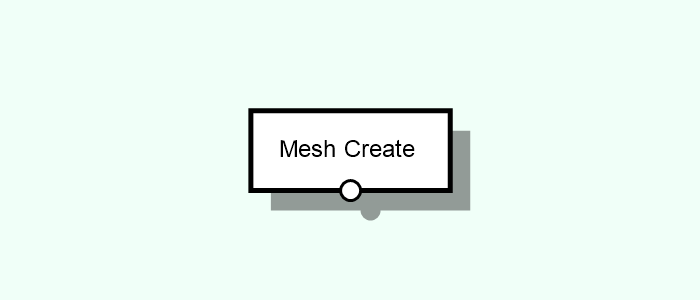# Mesh Create

Creates Meshes of primitive models (cubes, spheres), or from other Entity types.Tags: Mesh, Box, Circle, Cone, Cross, Cube, Cylinder, Dodecahedron, Face, From Surface, Icosahedron, L-Shape, From Path, Octahedron, Random Face, Rectangle, Sphere, Star, Text, T-Shape, U-Shape, Z-Shape

## Parameters#

Primitive [Select]
Type of primitive to create.
Box [Compound]
Creates a box.
Width [Float]
Width (size in X) of the box.
Length [Float]
Length (size in Y) of the box.
Height [Float]
Height (size in Z) of the box.
Circle [Compound]
Creates a circular shape.
Segments [Int]
Number of segments of the circle.
Cone [Compound]
Creates a cone.
Height [Float]
Height of the cone.
Segments [Int]
Number of horizontal segments (meridians) of the cone.
Cross [Compound]
Creates an cross shape.
Width [Float]
Width (size in X) of the shape.
Height [Float]
Height (size in Y) of the shape.
Body Width [Float]
Width (size in X) of the shape body. Should be smaller than the Width.
Body Height [Float]
Height (size in Y) of the shape body. Should be smaller than the Height.
Cube [Compound]
Creates a cube.
Size [Float]
Size of each side of the cube.
Cylinder [Compound]
Creates a cylinder.
Height [Float]
Height of the cylinder.
Segments [Int]
Number of horizontal segments (meridians) of the cylinder.
Dodecahedron [Compound]
Creates an Dodecahedron.
Size [Float]
Side length of the Dodecahedron
Face [Compound]
Creates a face from a set of coordinates.
Boundary [List]
Points defining the boundary of the face. At least 3 points are required and the first and last point should differ.
Point [Vector3D]
Point of the boundary
From Surface [Compound] [Adds ports 1]
Creates a mesh from a given surface.
Icosahedron [Compound]
Creates an Icosahedron.
Size [Float]
Side length of the Icosahedron
L-Shape [Compound]
Creates an L-shape.
Width [Float]
Width (size in X) of the shape.
Height [Float]
Height (size in Y) of the shape.
Body Width [Float]
Width (size in X) of the shape body. Should be smaller than the Width.
Body Height [Float]
Height (size in Y) of the shape body. Should be smaller than the Height.
From Path [Compound] [Adds ports 2]
Creates a mesh from a path entity.
Close Insides [Boolean]
Indicates if the insides of the closed paths should be transformed into faces.
Width [Float]
The width of the face created for each edge. Can be set as an expression based on the attributes of each edge using the @@attributeName notation.
Section [Attribute]
Attribute that will store the name the name of the section in the face. Values can be "Inside", "Joint" or "Path" and can be extracted with a "Mesh Subselect" node.
Octahedron [Compound]
Creates an Octahedron.
Random Face [Compound]
Creates a random looking face.
Seed [Int]
Seed of the random generator.
Size [Float]
Size/scale of the face.
Number of vertices [Int]
Number of vertices of the face.
Rectangle [Compound]
Creates a rectangle.
Width [Float]
Width (size in X) of the rectangle.
Height [Float]
Height (size in Y) of the rectangle.
Sphere [Compound]
Creates a sphere.
Horizontal Segments [Int]
Number of horizontal segments (parallels) in which the sphere will be divided.
Vertical Segments [Int]
Number of vertical segments (meridians) in which the sphere will be divided.
Star [Compound]
Creates a star shape.
Points [Int]
Number of segments of the star.
Radius of the first layer of points.
Radius of the second layer of points.
Text [Compound]
Creates a set of faces with the shape of a given input text.
Text [String]
Text Content.
Font [Choice]
Font of the text.
Size [Int]
Size of the text.
Style [Choice]
Font style.
Index [Attribute]
Stores the index of character in each face. The actual caracter can then be obtained in an expression from the original text.
T-Shape [Compound]
Creates an T-shape.
Width [Float]
Width (size in X) of the shape.
Height [Float]
Height (size in Y) of the shape.
Body Width [Float]
Width (size in X) of the shape body. Should be smaller than the Width.
Body Height [Float]
Height (size in Y) of the shape body. Should be smaller than the Height.
U-Shape [Compound]
Creates an U-shape.
Width [Float]
Width (size in X) of the shape.
Height [Float]
Height (size in Y) of the shape.
Body Width [Float]
Width (size in X) of the shape body. Should be smaller than the Width.
Body Height [Float]
Height (size in Y) of the shape body. Should be smaller than the Height.
Z-Shape [Compound]
Creates an Z-shape.
Width [Float]
Width (size in X) of the shape.
Height [Float]
Height (size in Y) of the shape.
Body Width [Float]
Width (size in X) of the shape body. Should be smaller than the Width.
Body Height [Float]
Height (size in Y) of the shape body. Should be smaller than the Height.

## Inputs#

This node has no native inputs.

### Parameter Inputs#

•  Input [Single | Surface]: Surface to be transformed to a mesh.
•  Input [Single | Path]: Path entity to be transformed into a mesh.

## Outputs#

• Output [Mesh]: Mesh created according to the defined parameters and/or inputs.